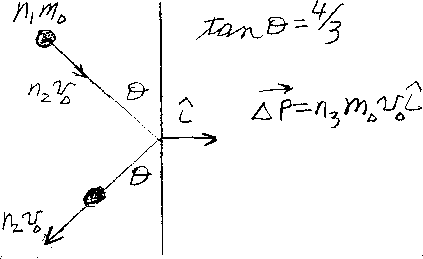Problem C2: An object of mass n1m0 is traveling with a speed of n2v0 towards a wall. The direction of its velocity makes an angle &theta with the wall, where tan &theta = 4/3. The object bounces elastically off the wall with the same speed and angle as shown in the figure above. If the change in momentum of the object is &Delta P = n3m0v0 i, What is n3? Note that the unit vector i points to the right.n1 = n2 = Input n3:
If you are currently in my class, you can record your grade by entering your name and student ID number (without the leading zeros) below and clicking on "record grade".
 First Name = Last Name = ID = Problem: